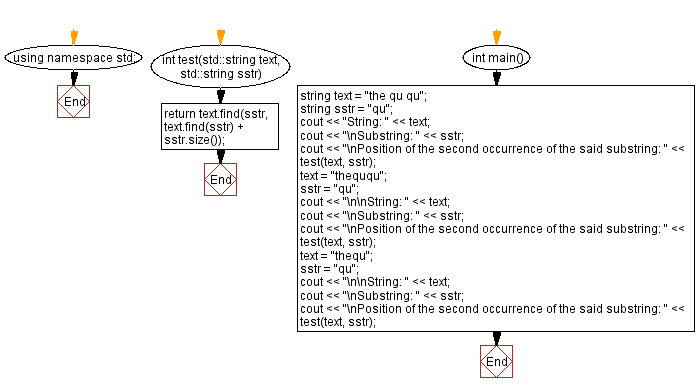﻿ C++ - Second occurrence of a string within another string

# C++ String Exercises: Second occurrence of a string within another string

## C++ String: Exercise-41 with Solution

Write a C++ program that finds the position of the second occurrence of a string in another string. If the substring does not appear at least twice return -1.

Test Data:
("the qu qu", "qu") -> 7
("theququ", "qu") -> 5
("thequ", "qu") -> -1

Sample Solution:

C++ Code:

``````#include <bits/stdc++.h>

using namespace std;

int test(std::string text, std::string sstr)
{
return text.find(sstr, text.find(sstr) + sstr.size());
}

int main()
{
string text = "the qu qu";
string sstr = "qu";
cout << "String: " << text;
cout << "\nSubstring: " << sstr;
cout << "\nPosition of the second occurrence of the said substring: " << test(text, sstr);
text = "theququ";
sstr = "qu";
cout << "\n\nString: " << text;
cout << "\nSubstring: " << sstr;
cout << "\nPosition of the second occurrence of the said substring: " << test(text, sstr);
text = "thequ";
sstr = "qu";
cout << "\n\nString: " << text;
cout << "\nSubstring: " << sstr;
cout << "\nPosition of the second occurrence of the said substring: " << test(text, sstr);

}
``````

Sample Output:

```String: the qu qu
Substring: qu
Position of the second occurrence of the said substring: 7

String: theququ
Substring: qu
Position of the second occurrence of the said substring: 5

String: thequ
Substring: qu
Position of the second occurrence of the said substring: -1
```

Flowchart:C++ Code Editor:

Previous C++ Exercise: Select lowercase characters from the other string.

Next C++ Exercise: Alternate the case of each letter in a string.

What is the difficulty level of this exercise?

﻿

## C++ Programming: Tips of the Day

Why does the C++ map type argument require an empty constructor when using []?

This issue comes with operator[]. Quote from SGI documentation:

data_type&operator[](constkey_type& k) - Returns a reference to the object that is associated with a particular key. If the map does not already contain such an object, operator[] inserts the default object data_type().

If you don't have default constructor you can use insert/find functions. Following example works fine:

```myMap.insert(std::map<int, MyClass>::value_type ( 1, MyClass(1) ) );
myMap.find( 1 )->second;
```

Ref: https://bit.ly/3PQBRJi

We are closing our Disqus commenting system for some maintenanace issues. You may write to us at reach[at]yahoo[dot]com or visit us at Facebook